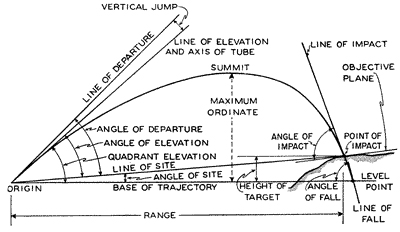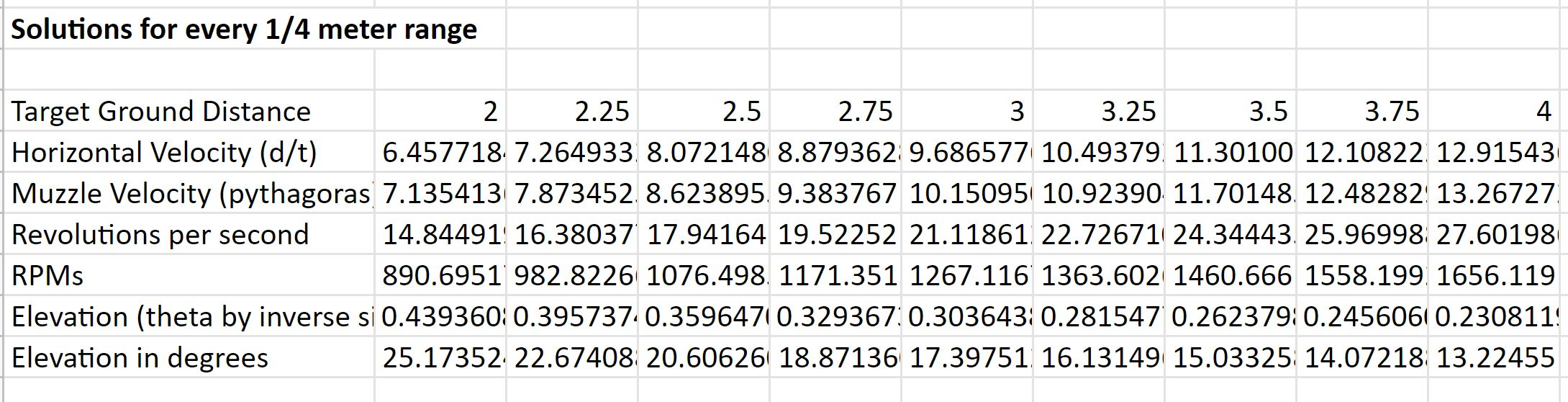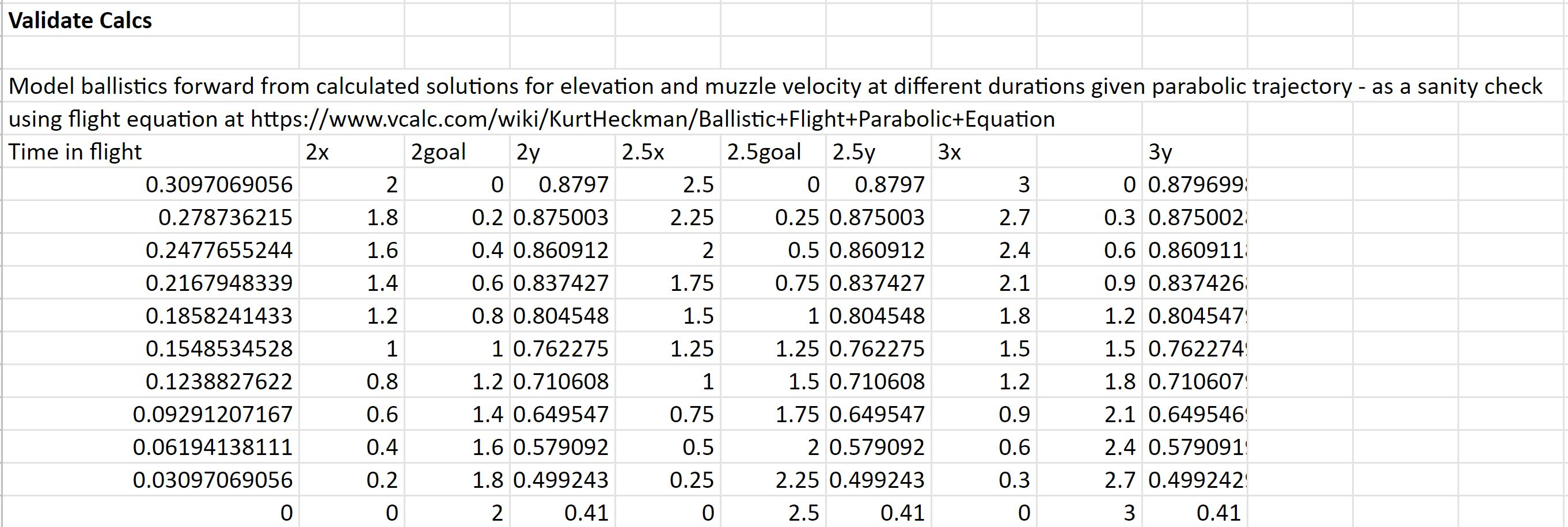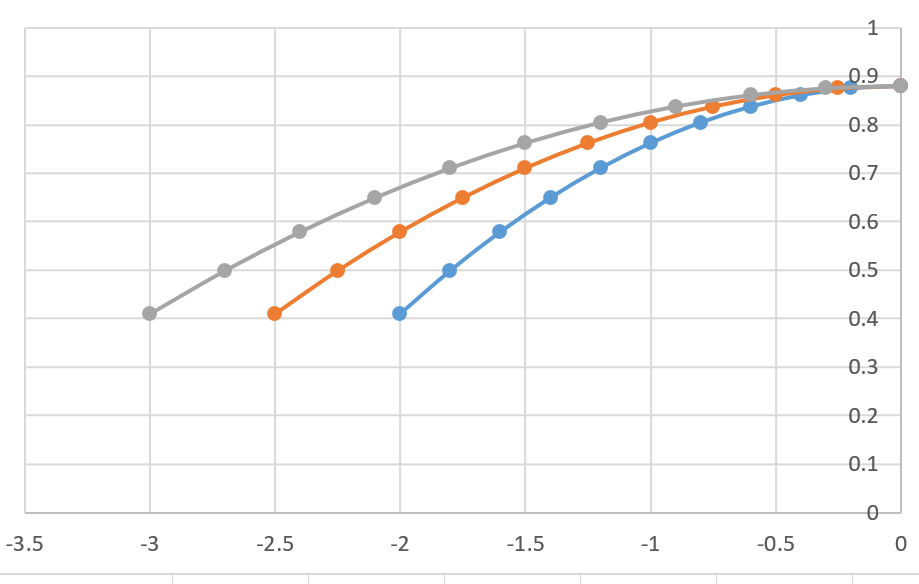# Correcting the Trajectory Calculations Equations

Tags: think and control
Personhours: 12
Correcting the Trajectory Calculations Equations By Bhanaviya, Ben, and Mahesh

### Task: Correct the trajectory calculations after the DPRG meetingIn the past week, we've been experimenting with a series of equations to derive the angle of launch of our flywheel launcher when we need it to travel a certain distance. 2 days ago, we were able to present the calculations we'd derived so far to Dallas Personal Robotics Group. After feedback from DPRG and a little more testing ourselves, we've discovered the following corrections we need to make to our equation. For reference, this is a correctional post regarding our earlier calculations - you can find versions of our earlier equations and calculations in our previous posts.

#### Time

One of the biggest fixes we needed to make to our equation was identifying how to derive time as a fixed variable. We wanted to be able to find the exact time in seconds it would take for our launcher to launch a ring at 0 vertical velocity. We needed this because we knew that for any shot that crosses through the goal with zero vertical speed, the ring needed to have an initial upward velocity such that the acceleration due to gravity brings it to zero vertical velocity at the point it reaches our target height. Regardless of what the horizontal component is, target time in this situation is governed by solely by gravity and vertical distance. As such, finding time at 0 vertical velocity would allow us to model an equation for the summit of the ring's trajectory, which is where we expect the goal post to be in order to reduce variability and error in our calculations. This understanding allowed us to create the following equation for time with h representing the height of vertical travel:

$\displaylines{t = \sqrt{h/(0.5* g)}}$

#### Initial Velocity

Initially, we had planned on solving for the initial vertical velocity of the ring then plugging into the equation for horizontal vvelocity, work backwards and find angle of launch. However, in order to have the most accurate trajectory possible, we expect the horizontal and vertical velocity to be varying, especially since the vertical velocity is affected by gravity and the horizontal isn't. Thus, we expect the vertical and horizontal initial velocity to look like the following, wherein t is time, initial vertical velocity is v_0 and initial horizontal velocity is h_v0:

$\displaylines{v_0 = g*t \\ h_v0 = d/t}$

#### Muzzle Velocity

Initially, we had planned on using velocity on its own for muzzle velocity. However, now that we have two initial velocities, our equation for muzzle velocity changed to look sort of like the Pythogorean Theorem with us solving for c, since the muzzle velocity represents the "hypotenuse" or trajectory in this case, mV representing muzzle velocity.

$\displaylines{mV = \sqrt{v_0^2 + h_v0^2}}$

### Initial Height of Launch

Previously, we measured the height of the robot - 0.41m - to be our initial height of launch. However, one issue with the vertical travel is that if we take 0.41 to be a variable which is affected by $$theta$$ then we need to figure out how much that change is for the vertical travel distance - an error bar or the design of experiments chart might work well for this so we can make sure angle of launch doesn't confound our initial measures for time and muzzle velocity since both of which rely on 0.47m (the height of travel taken by subtracting the height of the robot from the height of the goal) being constant which in turn relies on 0.41m.

#### Fixed Values

Since we know the height of travel to be 0.47m and $$g$$ to be 9.8 m/s^2, using our time equation, we found time to be 0.31s. Using time, we could then find the initial vertical velocity to be 3.055m/s and horizontal velocity dependent on the distance of the robot away from the robot, which we take to be our explanatory variable.

Our equation ultimately stays the same but the methods we used to calculate time and initial velocities have now changed, which should change our final values substantially. In our latest post, we had a set of very different values in our meeting with DPRG. However, with these edits, we now have the following calculations (which we simplified by plugging into a spreadsheet to get automated values.In order to sanity-check our calculations, we also derived the following parabola to represent the arc of the trajectory:### Next Steps:

Our immediate next steps are to model initial height as a function of $$theta$$. Since we know that initial height is susceptible to change as the elbow of the robot drops and raises in accordance with angle of launch, being able to model initial height as a function will allow us to reduce all possible sources of error and find an accurate measurement of trajectory calculations. This will likely require another process like the one we did here as we attempt to model initial height as a function. W'd like to thank DPRG for all their feedback and for their help in allowing us to fine-tune these calculations!

Date | January 28, 2021